# Line Voltage to Phase Voltage Line Current to Phase Current Relationship

##### Update:

In a three-phase balanced system, the voltage across the phase with respect to another phase is always equal in the magnitude of the voltage and phase angle and the vector sum of the three-phase is always zero.

The line voltage or phase voltage above 440Volts can be measured with the help of a potential transformer. Potential meter reduces the voltage from a higher level to low level typically 110 Volts to 63.5Volts.

At the same time the line current or phase current above 25Amps, Current transformer is used to reduce the current level from high to low typically 1A or 5A.

## What is Line Voltage:

In a three-phase power system, The potential difference between the two phases is called line voltage (typically phase to phase). It is denoted by VL-L . The voltage between R to Y or Y to B or B to R. In a power system, system voltage means line to line voltage. Refer to the Diagram,

Learn More:   Why Slip ring Induction motor is preferred for High starting Torque application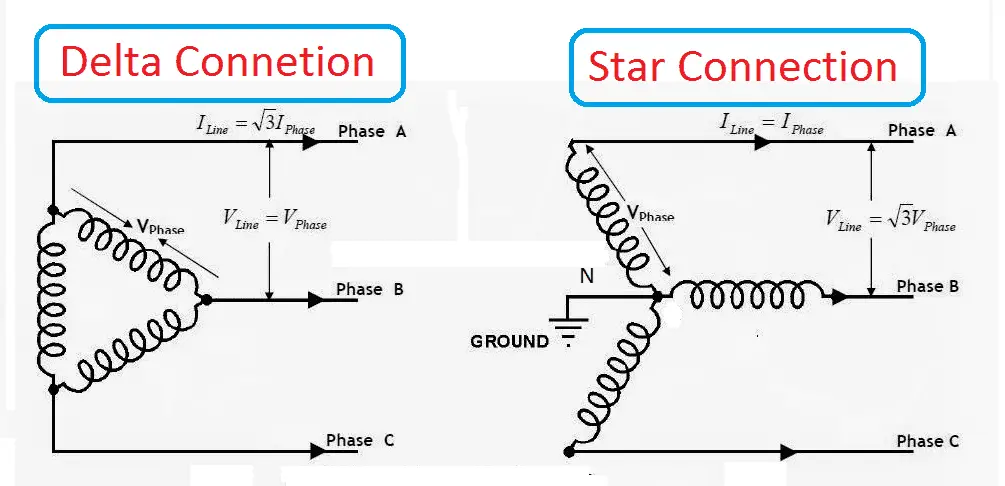Example: our domestic power supply is three phases, 440 Volts. Here 440 volts means the phase to phase voltage is 440.

Note: If they mention in single phase 230 volts, then it means the potential difference the phase to neutral is 230 volts.

In star connection: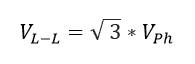Line voltage = 1.732 times of phase voltage.

In delta connection: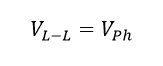Line voltage = Phase voltage.

## What is line current:

The measure of current in one phase before the star or delta arrangement of the component is called line current (typically input current in motor or output current in the alternator). In three phase balanced system, it may be R phase current or Y phase current or B phase current.

It is denoted by IL ampere.

In star connection: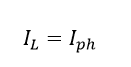Line current= phase current. (we get it from applying Kirchhoff’s current rule.)

In delta connection: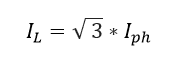Line current= phase current. (we get it from applying Kirchhoff’s voltage rule.)

## What is phase voltage:

In three phase system, the potential difference between one phase to natural point is called phase voltage. It is denoted by Vph volts

Star Connection: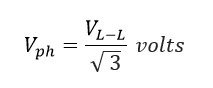Phase voltage = Line voltage divide by 1.732

In Delta Connection:## Phase current:

Phase current is the measure of the current inside of star connection or delta connection of the three phase system. It is denoted by Iph.

In star connection: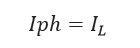Phase current = Line current

In delta connection: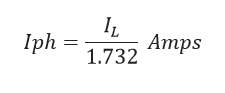Note: Value of3 = 1.732.